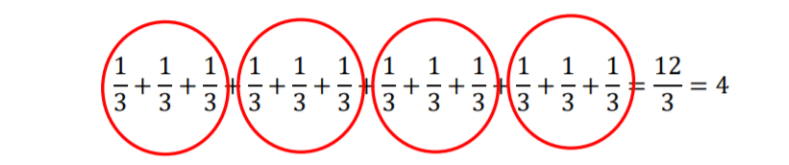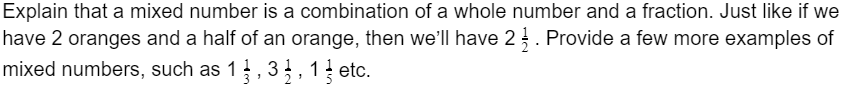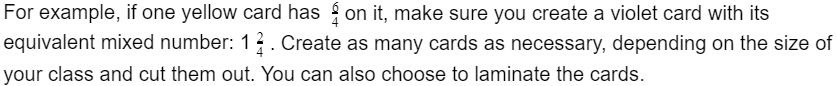# Understanding Fractions as Division

 Listen to this Lesson:

When learning about fractions, fifth graders learn how to interpret fractions as division of the numerator and denominator, model fractions as division by using a tape diagram, as well as solve word problems involving fractions as division.

If you’re a math teacher and you’re teaching fractions as division, you’re probably familiar with the fact that this is not the most fun topic for children! However, with the right guidelines and activities, you can make your lesson as inviting as it gets! Read on to learn more.

## Guidelines to Teach Fractions as Division

### What Are Fractions?

You can start your lesson on fractions as division by defining fractions. Point out that we use fractions to represent a part of a whole. Each fraction has a numerator and a denominator. The numerator is the top number, whereas the denominator is the bottom number.

Add that the horizontal line that separates this top and bottom number is called a ‘fraction bar’.

Present a drawing of a fraction on the whiteboard, and illustrate the numerator and denominator in it.

For instance, you can draw a pizza circle and divide it into 5 equal pizza slices. Illustrate 2⁄5 by shading two parts in the pizza drawing. Highlight that 2 is the numerator in this fraction, and 5 is the denominator.### Interpreting Fractions as Division

Explain that a fraction is a number written like a division problem. A fraction is the division of the numerator by the denominator. Provide a drawing on the whiteboard that could illustrate how fractions can be expressed as division, such as the following drawing:Provide a few examples of fractions that are common so that students can grasp fractions as division:

• 1⁄2 is the same as 1 ÷ 2
• 2⁄3 is the same as 2 ÷ 3
• 3⁄4 is the same as 3 ÷ 4

To think of fractions as division problems may come across as strange, particularly since, in these kinds of division problems, we divide smaller numbers by larger ones. This, however, is possible, it just means that the quotient we’ll get is smaller than one.

### The Unit Form of Fractions

Now that students know that a fraction is the division of the numerator and the denominator, you should also illustrate how we write the unit form of fractions. For example, let’s say we want to write the division problem 4 ÷ 3 as a fraction and then write the fraction in its unit form.

Writing a division problem as a fraction should be easy for students by now. But writing a fraction in its unit form is more challenging. How can we do this? First, point out that in 4 ÷ 3, we’re dividing 4 whole into 3 equal parts.

Add that there are 12 one-thirds (1⁄3) in 4. Explain that 12 one-thirds is the same as twelve-thirds or 12⁄3. You can also illustrate this on the whiteboard so that students can visualize it better:Thus, 4 ÷ 3 = twelve thirds ÷ 3 = four thirds = 4⁄3.

### Types of Fractions

Point out that there are several types of fractions, including proper fractions, improper fractions, and mixed numbers. Define each of these three types and provide examples.

#### Proper Fractions

Explain that proper fractions are the fractions that most often think of when we imagine a fraction. In other words, these are fractions where the numerator is smaller than the denominator. Provide a few examples, such as 2⁄3, 1⁄2, 3⁄5, 4⁄5 etc.

#### Improper Fractions

Explain to students that an improper fraction is a fraction whose numerator is greater than the denominator. Provide a few examples of improper fractions, such as 4⁄3, 7⁄2, 6⁄5, 8⁄7 etc.

#### Mixed Numbers### Changing an Improper Fraction Into a Mixed NumberIf you have the technical means in your classroom, you can also enrich your lesson on fractions as division with multimedia materials, such as videos.

For example, this video provides a good introduction to fractions as division. Then, play this video to explain how we can use a tape diagram to model fractions as division. Finally, use this video to illustrate the process of changing an improper fraction into a mixed number.## Activities to Practice Fractions as Division

### ‘I Have…Do You Have?’ Game

This is an exciting game where students will get to move around the classroom and practice their knowledge of mixed fractions and improper fractions. To implement this game in your classroom, you’ll need yellow and violet construction paper, some scissors and markers.

Create cards on the construction paper, and write an improper fraction or a mixed number on each card. If there is an improper fraction on the yellow paper, make sure there is an equivalent mixed number on the violet paper.Divide the students into two bigger groups and hand out the cards to each group. Distribute the yellow cards to one group and the violet cards to the other group, making sure that each student has received one card.

Provide instructions for the game. Explain that this is a matching game, where students go around the room and try to find the other student that holds a card with an equivalent value. When approaching a student, they ask: ‘I have card x, do you have card y..?’

This way, students practice converting improper fractions to mixed numbers and vice versa.

Allow a few minutes for students to complete the game and find their match. In the end, provide time for questions and discussion.

### Fractions as Division Online Game

In this simple online game, students will get to practice interpreting fractions as division. Use this game in your classroom at the end of your lesson on fractions as division. The only resource needed is a suitable device for each student.

In each exercise, students are presented with multiple answers where they have to select the correct numerical expression that is equal to the given fraction or select the right fraction that is equal to the given expression, by relying on their knowledge of fractions as division.

Students play the game individually. They can also use a hint or play a related video with instructions on understanding fractions as division if they get stuck on a problem that they should work out.

### Mixed Fraction Maze

This is an engaging online game that provides students with the opportunity to practice changing mixed fractions into improper fractions. The only materials needed to use this game in your classroom are enough technical devices.

Provide instructions for the game. Explain that there is a deadly scorpion in the game that is searching for food. Players have to help the scorpion eat in the maze and feed it, however, the scorpion must only eat certain bugs.

Students are presented with questions where they have to answer how to convert a given mixed number into an improper fraction. The bugs are of different colors and it is in them that the answers are contained. By selecting the bug with the right answer, students feed the scorpion.

Students play the game individually, which makes it ideal for homeschooling parents as well. At the end of the game, open space for discussion and reflection. How did students identify which mixed numbers equal which improper fractions?

### Fractions to Mixed Numbers Game

In this interactive online game, students practice converting improper fractions to mixed numbers. To use this game in your classroom, make sure you have enough suitable devices for each student.

Divide students into pairs and provide instructions. Students are presented with different exercises where they have to convert a given improper fraction to a mixed number. If they answer correctly, they score points.

In the end, players in each pair compare their final scores. The person with the highest score is declared the winner. Parents who are homeschooling their children can easily adapt the game to an individual game.

## Before You Leave…

If you enjoyed these ideas and activities for teaching fractions as division, you’ll want to check out our lesson that’s dedicated to this topic! So if you need guidance to structure your class and teach it, sign up for our emails to receive loads of free content!

Feel free to also check out our blog – you’ll find plenty of awesome resources that you can use in your class! And if you’re ready to become a member, simply join our Math Teacher Coach community!